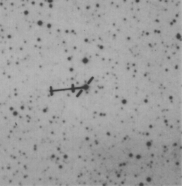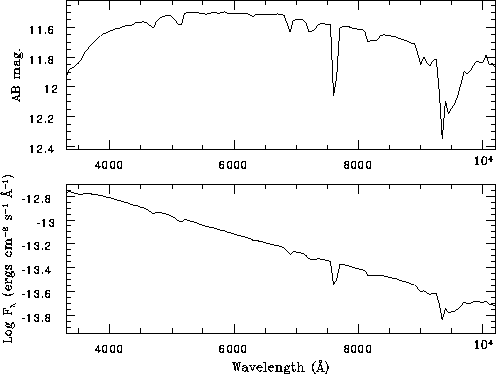# LTT 4364

alpha(2000) = 11h 45m 42.92s , delta(2000) = -64d 50' 29.5''
Proper motion (Δα, Δδ) = (2665, 296) milli-arcseconds per year. Coordinate epoch 2000.

V = 11.50, B-V = +0.16, Spectral type: C2

``

Field is 7.0 x 7.0 arcmin

``

Hamuy et al (1992, 1994) data (50A bins)

ftp access to visible wavelength data files for this standard